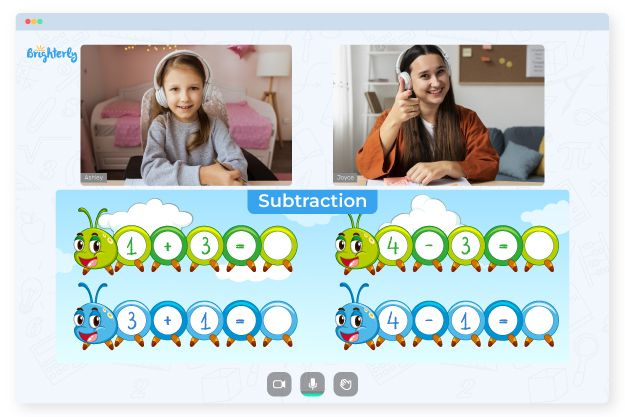# Subtraction With Borrowing Worksheets

An in-depth understanding of subtraction will give children clear understanding on how to borrow numbers to make another number bigger. Math questions can be tough for kids if they don’t have a proper guide. Here is how subtraction with borrowing worksheets can help them in that regard.

## Why Do Tutors from Brighterly Use Subtraction with Borrowing Worksheets?

Brighterly is an online platform with tutors that teach students how to handle math problems. When subtracting big numbers, a column’s top digit may be smaller than a bottom digit. So, Brighterly’s tutors use borrowing subtraction worksheets to show kids what to do in that scenario and better understand subtraction.

Also, subtraction borrowing worksheets help kids understand borrowing. They learn to subtract 1 from the borrowing number, and then put the result above the number they crossed out.

Tutors advise students to borrow from the tens column while subtracting in the units column. And to handle the tens aspect, they can borrow from the hundreds. Subtraction worksheets with borrowing usually start with small figures before offering operations with higher ones.

Math for Kids

Is Your Child Struggling With Math?
1:1 Online Math Tutoring## Subtraction with Borrowing Worksheets: An Exciting Way to Learn

When subtracting a more significant number from a smaller one, when a subtrahend is greater than a minuend, you borrow. You don’t need a subtraction borrowing from zero worksheet for simple equations like 26 – 14 since 6 (the minuend) is higher than 4 (the subtrahend). But when it comes to 50 – 13, it is impossible to subtract 3 from 0, so borrowing will be necessary.### Subtraction With Borrowing Worksheets PDF

Borrowing Subtraction Worksheets### Subtraction With Borrowing Worksheets PDF

Subtraction Borrowing Worksheets### Subtraction With Borrowing Worksheets PDF

Subtraction Worksheets With Borrowing### Subtraction With Borrowing Worksheets PDF

Subtraction With Borrowing Worksheet

Children can learn how to borrow figures during subtraction by studying subtraction workbooks offered by their parents and instructors. But a subtraction with borrowing worksheet will teach children those concepts in an engaging manner. In addition, worksheets can significantly enhance kids’ problem-solving and decision-making abilities. So, to assist your child in learning how to subtract numbers with a lower minuend, get a worksheet today.

### Subtraction Worksheets

Trouble with Subtraction?• Is your child finding subtraction more challenging than expected?
• Start lessons with an online tutor.

Does your child struggle to understand subtraction? Try studying with an online tutor.